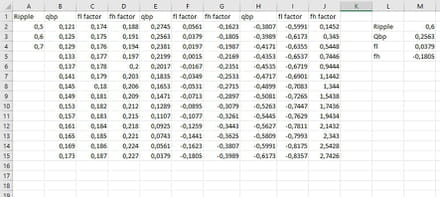# Change cell list values when another cell is changed

Solved/Closed
Rolnaashch - Jun 24, 2021 at 09:34 AM
Rolnaashch - Jul 5, 2021 at 04:43 PM
Hello,

My calculations would go a bit further, and i don't really know if my issue can be solved by excel.
I have multiple lists for speaker box designing. And i try to make an easier calculation for calc the box parameters by just selecting values from the dropdown list, since it's predefined.

So there are 3 main "ripple effects" by values of 0.7, 0.6, 0.5.
Then for example someone would select 0.6, then in another cell, which has a dropdown list with 17 values will change depending on the main cell. So basically for 0.7 main values has 17 different sub-values, and the other two as well.

Is this possible to make?

Thank you for the help in advance!

System Configuration: Windows / Chrome 91.0.4472.114

## 6 replies

TrowaD Posts 2921 Registration date Sunday September 12, 2010 Status Moderator Last seen January 16, 2023 547
Jun 29, 2021 at 12:15 PM
Hi Rolnaashch,

You can do so by creating named ranges and then use the function INDIRECT to refer to them.
In your case you also need the function IF, since the numerical values you use, can't be used for named ranges.

Let us know if you need further assistance.

Best regards,
Trowa
Thank you very much for your help.
It appears i migh bite more than what i can chew here. :D

Because my finale goal would be the same method but on multiple structures.

Writing a program in delphy would be probably more effective and seemingly easier. I cannot tell.

Here is this table, where there are 3 main values.

https://images.audiojudgement.com/2016/03/4th-order-bandpass-ripple.jpg

The first dropdown list would include the 3 main S values.

Then fog eg. the first value has a list of Qbp values. So those Qbp values should load into a dorpdown list array again. So selecting the proper Qbp would load into different cells fL factor, and fH factor.

But yet again, i think i have to think about this way much more and longer time to learn and understand the possibilities.

Once again, much appretiation for the guiding help and time!

Best Regards,
-Roland
TrowaD Posts 2921 Registration date Sunday September 12, 2010 Status Moderator Last seen January 16, 2023 547
Jul 1, 2021 at 12:06 PM
Hi Roland,

From your table I don't see how selecting a Qbp value would result in a different selection of fL factors.

Wouldn't selecting a S value of 0.7 result in the following 3 dropdown lists?
The first being Qbp with the 14 values from 0.4507 to 1.1321
The second being fL with the 14 values from 0.2167 to 0.8277
The third being fH with the 14 values from 0.9373 to 1.5483

When selecting a S value of 0.6, then those dropdown lists would change.
The first being Qbp with the 14 values from 0.5258 to 1.3207
The second being fL with the 14 values from 0.2326 to 0.9266
The third being fH with the 14 values from 1.1886 to 1.8826

Isn't this what you are looking for, or am I missing something?

Best regards,
Trowa
Hello Trowa,

Thank you again for your kind reply and the time to take a look at my issue/plan.

Well, the idea would be the following:

There are two values which need to be determined depending on "S" and "Qbp" values.

fL is the low frequency roll-off, and fH is the high frequency roll-off.
The formulas to calculate those are these:

fL = Fs / Qts * (fL factor)
fH = Fs / Qts * (fH factor)
[Fs and Qts is given by the manufacturer of the speaker]

So there would be 2 dropdown list. One is "S" and the other is "Qbp".

For example if i would select "S=0,6" then the "Qbp" list would start with the "0,5258" - column and below.
Then selecting a value from the "Qbp" dropdown would give an automatic value to "fL factor" and "fH factor" cells. So making another automated calculations for other values as well. But these values are the bases for almost all the parameters.

Thank you for your time, but i don't want to stress this out too much, since i don't know if this would be possible at all.

Didn't find the answer you are looking for?

TrowaD Posts 2921 Registration date Sunday September 12, 2010 Status Moderator Last seen January 16, 2023 547
Updated on Jul 5, 2021 at 12:28 PM
Hi Roland,

It is definitely possible!

This is how I set up your sample data:The results are in columns L and M.

Ripple result in M2:
I named range "A2:A4" ripple and then used the formula:=INDIRECT("ripple") in data validation.

Qbp result in M3:
I named range "B2:B15" qbpl7
I named range "E2:E15" qbpl6
I named range "H2:H15" qbpl5
Then in data validation used the formula:
=IF(\$M\$2=0.7,INDIRECT("qbpl7"),IF(\$M\$2=0.6,INDIRECT("qbpl6"),IF(\$M\$2=0.5,INDIRECT("qbpl5"))))

fl result in M4:
Use the formula:
=IF(\$M\$2=0.5,VLOOKUP(\$M\$3,\$H\$2:\$J\$15,2,0),IF(\$M\$2=0.6,VLOOKUP(\$M\$3,\$E\$2:\$G\$15,2,0),IF(\$M\$2=0.7,VLOOKUP(\$M\$3,\$B\$2:\$D\$15,2,0))))

fh result in M5:
=IF(\$M\$2=0.5,VLOOKUP(\$M\$3,\$H\$2:\$J\$15,3,0),IF(\$M\$2=0.6,VLOOKUP(\$M\$3,\$E\$2:\$G\$15,3,0),IF(\$M\$2=0.7,VLOOKUP(\$M\$3,\$B\$2:\$D\$15,3,0))))

That should do it.

Here is my test file for reference: